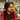## Properties of a Linked List:

• A linked list is a data structure which consists of ‘nodes’ linked together.
• Each ‘node’ has two fields/properties:

1. Some data/value
2. A link to the next node

``````var ListNode = function (val) {
this.val = val
this.next = null
}``````

``````var root = new ListNode(1)
root.next = new ListNode(2)
root.next.next = new ListNode(3)
root.next.next.next = new ListNode(4)

// Above linked list: 1 -> 2 -> 3 -> 4``````

## Constructing a Linked List from an array:

``````var createListFromArray = function (array) {
var root = new ListNode(0) //Dummy value. Used to remember where the linked list actually starts from.
var curr = root

for (var i = 0; i < array.length; i++) {
curr.next = new ListNode(array[i])
curr = curr.next
}

var startOfList = root.next
}

var arr = [1, 2, 3, 4, 5]
var list = createListFromArray(arr)
// Above linked list: 1 -> 2 -> 3 -> 4 -> 5``````

``````//Lets try to print the linked list created above
var list = createListFromArray(arr)

var printList = function (listHead) {